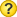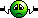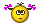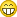# i need a FFT routine for bascom avr , atmega128

This site uses cookies. By continuing to browse this site, you are agreeing to our Cookie Policy.

• # i need a FFT routine for bascom avr , atmega128

Hi folks,
I am new here end looking for a peace of program for a FFT calculation.

please also the dimensioning of the variables.

i do have the routine for purebasic, but bascom does not accept the variables in
the same way as bascom does. So i can not get the program working.

i do use bascom 2.0 7.8

i like to incorperate this routine in my normal bascom programs.

thank you very much

Wim PA0SLT
• And the question isWhat we can do without your Code?The easiest way is coyp all into Bascom and correct all the compiler is'nt lucky about• FFT (fast Fourier transform)
Files
Code first, think later - Natural programmer• Hallo wimapon,

perhaps you may have a look to my FFT-program, but the info-Text is in german.

### BASCOM Source Code: FFT

1. '( FFT-Programm für Frequenzen bis 8000Hz
2. ohne Verwendung von Assembler-Routinen
3. 11.02.2019 /18:47
4. jedoch ist Overclocking des ATmega644 mit 25MHz, möglich, womit damit die Dauer
5. der FFT-Berechnung minimiert werden kann.
6. Wegen des relativ geringen RAM-Speichers des ATmega644 ist die FFT auf
7. 128-Punkte begrenzt.
8. Frequenzen bis max. 8000Hz bei 128 Abtastpunkten bei 16000Hz Abtastfrequenz
9. Frequenzauflösung : 16000Hz / 128 = 125 Hz Pro Filter
10. Programm-Schritte:
11. 0) Berechnung der Sin/Cos-Werte in einem vorgelagerten Schritt (Wsin(ptr) und Wcos(ptr))
12. 1) Einlesen von 128 Werten mittels GETADC(0) in Re(i) (Amplituden: 0V bis 2,56)
13. 2) Durchführen der FFT über 128 Punkte
14. 3) Ausgabe von 64 FFT Werten in Re_betrag(k) über DA-Wandler, alternativ via Print-Command
15. Der Daten-Einlesezyklus wird von Timer1 (via Interrupt) in festen Zeitabständen
16. gestartet. Während der FFT-Runtime wird der Timer1-Interrupt abgeschaltet, da
17. FFT-Berechnung länger als der Daten-Einlesezyklus dauert.
18. Bei der Datenausgabe via DA-Wandler kann ein separater fester Timer0-Zyklus
19. aktiviert werden, um die Dauer der analogen Amplituden-Darstellung den Erfordernissen anzupassen.
20. Es sollte ein Antialias Filter vorgeschaltet werden, um Faltungsprodukte
21. zu verhindern.
22. Es ist sinnvoll, für langsamere Eingangsfrequenzen einen längeren Timer1-Zyklus
23. (z.B. prescaler=8) zu wählen, damit bei niedrigen Frequenzen genügend Abtastwerte
24. pro Schwingung erfaßt werden können.
25. Da der Timer1 bis zum Überlauf/Ende = 2^16= 65536 zählt, sind für die
26. gewünschten Zeiten (65536 - berechneter Wert) in den Timer1_preload zu setzen.
27. ')
28. \$regfile = "m644def.dat"
29. \$crystal = 20000000 '20MHz
30. '\$crystal = 25000000 '25MHz!!!! 5MHz Overclocked funktioniert
31. \$hwstack = 100
32. \$swstack = 100
33. \$framesize = 100
34. '\$sim
35. \$baud = 56000
36. Dim Sample As Word 'ADC Sample
37. Dim Re(130) As Single 'Real-Teil vor FFT-Berechnung
38. Dim Im(130) As Single 'Imag-Teil vor FFT-Berechnung
39. Dim Real(130) As Word 'Betragswerte nach FFT-Berechnung
40. Dim Wcos(130) As Single 'Cosinus Werte für FFT
41. Dim Wsin(130) As Single 'Sinus Werte für FFT
42. Dim Pi As Single
43. Dim Mr As Integer
44. Dim N As Word 'FFT-Punkteanzahl
45. Dim Nn As Integer
46. Dim L As Integer
47. Dim M As Integer
48. Dim Tr As Single
49. Dim Ti As Single
50. Dim Istep As Integer
51. Dim A As Single
52. Dim Wr As Single
53. Dim Wi As Single
54. Dim I As Integer
55. Dim J As Integer
56. Dim Tr0 As Single
57. Dim Tr1 As Single
58. Dim Ti0 As Single
59. Dim Ti1 As Single
60. Dim Nm As Integer
61. Dim W_cond As Integer
62. Dim Ptr As Integer
63. Dim Re1 As Single
64. Dim Im1 As Single
65. Dim Re_betrag As Single
68. Dim Flag_t0 As Byte
69. Dim Flag_t1 As Byte
70. Dim K As Integer
71. Dim N2 As Byte 'FFT-Ergebnis, N-halbe Slots
72. Dim N2_1 As Byte 'N-halbe +1
73. Dim Freq As Word 'Frequenzvorgabe für Testsinus
74. Dim Abtast_dauer As Single 'Abtastdauer für Testsinus
75. 'Dim Sin_input(128) As Single 'Speicherplatz für Testsinus
76. Dim F_steps As Single 'Frequenzsteps für Testsinus
78. Dim Schritt As Single 'Schrittweite für Testsinus
79. Dim Sinuswert As Single
80. Dim Nom_preload As Word 'Abtastdauer = 1/f_Abtast, z.B 1/8000Hz = 125us Abtastdauer
81. Dim Isr_korrektur As Word 'ISR-Routinen Korrektur
82. Dim Da_config_bits As Word
83. 'Hinweis zur ISR-Routinen Korrektur: Dauer ~3.2us. Die ISR-Routine benötigt u.a.
84. 'extra Quarz-Takte,um die Rücksprung-Adressen zu sichern, wodurch sich die berechnete
85. 'Abtastdauer verlängert. Durch diese Korrektur passen die ermittelten FFT-Frequenzen zu
86. 'den FFT-Slot-Nummern.(1-64)
87. 'z.B. Abtastrate=8000Hz, bei 128 FFT-Punkten ergib dies 8000/128=62.5Hz pro FFT-Slot
88. 'Beispiel: Bei einer Eingangsfrequenz von 1995Hz erscheint diese Linie im
89. 'FFT-Slot 1995Hz/62.5Hz = 32. Somit kann eine FFT-Linien-Zuordnung erfolgen.
90. 'Config Adc = Single , Prescaler = 32 , Reference = Internal_2.56
91. Config Adc = Free , Prescaler = 32 , Reference = Internal_2.56
93. Declare Sub Fft
94. '----- Config DA-Wandler
95. Config Porta.5 = Output
96. Da_sclk Alias Porta.5 'DA-Wandler LT1451 Clock
97. Config Porta.6 = Output
98. Da_data Alias Porta.6 'DA-Wandler LT1451 Daten
99. Config Porta.7 = Output
100. Da_cs Alias Porta.7 'DA-Wandler LT1451 CS
101. Config Portd.6 = Output 'Testport
102. Testport Alias Portd.6
103. '-- Timer1-Vorgabe für max. Abtast-Frequenz von z.B. 8000Hz -----
104. Config Timer1 = Timer , Prescale = 1
105. Enable Timer1
106. On Timer1 Isr_timer1
107. 'Nom_preload = 64276 'entspricht hier 1/15873Hz = 63us Abtastdauer
108. 'Isr_korrektur = 200 'Korrektur wegen ISR und ADC-Conversion Zeitverlust
109. Nom_preload = 63036 'entspricht hier 1/8000Hz = 125us Abtastdauer
110. Isr_korrektur = 116 'Korrektur wegen ISR und ADC-Conversion Zeitverlust
112. '----- Timer1-Vorgabe für max.Frequenz von ~200Hz (100Hz) ----
113. 'Config Timer1 = Timer , Prescale = 8
114. 'Enable Timer1
115. 'On Timer1 Isr_timer1
116. Flag_t0 = 0
117. Flag_t1 = 0
118. Freq = 1290 'Hz
119. Abtast_dauer = 0.000125 'Abtast-Takt, hier 125usec
120. F_steps = 1 / Freq 'Anzahl Steps pro Schwingung mit Abtastdauer
121. F_steps = F_steps / Abtast_dauer
122. Pi = 4 * Atn(1)
123. Step_radian = 2 * Pi 'Anzahl Steps pro Schwingung bezogen auf 1 Radian
126. '--------------------------------------------------------------
127. N = 128 'Anzahl Punkte der FFT
128. N2 = N / 2 'N-halbe
129. N2_1 = N2 + 1 'N-halbe + 1, wegen DA-Wert=0 nach N2
130. Schritt = 0
131. For I = 1 To N 'Generierung von Sinus-Werten zum Testen
132. 'Sinuswert = Sin(schritt)
133. 'Sin_input(i) = 400 * Sinuswert
134. 'Schritt = Schritt + Step_radian
135. 'Re(i) = Sin_input(i)
136. 'Im(i) = 0
137. Next
138. ' ------ Start -----------
139. 'Vorgelagerte Cos- und Sin- Berechnung
140. L = 1
141. Nm = N
142. While L < N '
143. Istep = 2 * L
144. For M = 1 To L
145. Ptr = M + L
146. A = 1 - M
147. A = A / L
148. A = A * Pi
149. A = A * -1 'A=-Pi*(1-M)/L
150. Wcos(ptr) = Cos(a)
151. Wsin(ptr) = Sin(a)
152. Wsin(ptr) = Wsin(ptr) * -1 '-sin(a)
153. 'Print "Ptr=" ; Ptr ; " Cos=" ; Wcos(ptr) ; " Sin=" ; Wsin(ptr)
154. Next M
155. L = Istep
156. Wend
158. Enable Interrupts
160. Start Timer1
161. I = 1
162. Do
163. Do 'warten auf Signal-Einlese-Schluß
164. nop
165. Loop Until Flag_t1 = 1
166. 'Reset Testport
167. Flag_t1 = 0
168. '
169. '--------FFT-Routine Zeitdauer-Messbereich Anfang ------------------------
170. ' (Dauer 66.4ms/128 Punkte/20MHz-Quarz)
171. ' (Dauer 53.2ms/128 Punkte/25MHz-Quarz)
172. '----FFT Routine
173. 'Set Testport
174. Fft
175. ' '----Betragsberechnung
176. For I = 1 To N2 '
177. Re1 = Re(i) * Re(i) 'Re(i) zum Quadrat
178. Im1 = Im(i) * Im(i)
179. Re_betrag = Re1 + Im1
180. Re_betrag = Sqr(re_betrag)
181. Re_betrag = Re_betrag / 4 'reduzieren, da Wert > als 65536 sein kann
182. Real(i) = Re_betrag 'Betragswert
183. Print I ; " " ; Real(i) 'Betragswert-Ausgabe der einzelnen FFT-Slots
184. Next I
185. Real(n2_1) = 0 'N-halbe+1 Wert auf Null gesetzt, für DA-Ausgabe
186. Real(1) = 0 '#####!!!!Gleichanteil zu Null gesetzt!
187. 'Reset Testport
188. '--------------------------- Zeitdauer-Messbereichs Ende ----------------------
189. ' --Start D/A-Wandlung , für Darstellung auf Oszilloskop - - - - - - - - - - -
190. Set Testport 'für Oszilloskop: Start-Trigger
191. Waitus 10
192. Reset Testport
193. Da_config_bits = &B0010000000000000
194. K = 0 'Start bei 1, um Gleichanteil auszublenden
195. Do
196. Incr K
197. Da_cs = 0
198. Shift Real(k) , Right , 1 'Amplitude für 12-bit DA_Wandler Output anpassen
199. Real(k) = Real(k) Or Da_config_bits
200. Shiftout Da_data , Da_sclk , Real(k) , 0
201. Da_cs = 1 'Cs=1=Ende DA-Daten Transfer
202. Waitus 180 'Darstellungsdauer pro Step
203. Loop Until K = N2_1 'nächsten Ausgabewert nach N-Halbe auf Null gesetzt
204. Enable Interrupts
205. I = 1
207. Start Timer1
208. Loop '######################################
209. End
210. Isr_timer1:
211. 'Set Testport 'Ermittlung der Abtastdauer mit Oszilloskop
213. Flag_t1 = 0
215. 'Reset Testport
216. 'Re(i) = Sin_input(i) - 400
217. Re(i) = Sample - 512 'Offset Befreiung
218. Im(i) = 0
219. Incr I
220. If I = 129 Then
221. Stop Timer1 '
222. Disable Interrupts
223. Flag_t1 = 1
224. End If
225. Return
226. Sub Fft
227. Mr = 0
228. Nn = N - 1
229. For M = 1 To Nn
230. L = N
231. L = L / 2 'Shift L , Right , 1 ' = L / 2
232. W_cond = Mr + L
233. While W_cond > Nn
234. L = L / 2 'Shift L , Right , 1 ' = L / 2
235. W_cond = Mr + L
236. Wend
237. Mr = Mr Mod L 'Mod_argu
238. Mr = Mr + L
239. 'Mr = Mr
240. If Mr > M Then
241. Tr = Re(m + 1)
242. Re(m + 1) = Re(mr + 1)
243. Re(mr + 1) = Tr
244. Ti = Im(m + 1)
245. Im(m + 1) = Im(mr + 1)
246. Im(mr + 1) = Ti
247. End If
248. Next M
249. L = 1
250. Nm = N
251. While L < N '
252. Istep = 2 * L
253. For M = 1 To L
254. Ptr = M + L
255. Wr = Wcos(ptr) 'cos(a)
256. Wi = Wsin(ptr) 'Sin(a)
257. For I = M To N Step Istep
258. J = I + L
259. Tr0 = Wr * Re(j)
260. Tr1 = Wi * Im(j)
261. Tr = Tr0 - Tr1 'ZR=CO*RE(J) - SI*IM(J)
262. Ti0 = Wr * Im(j)
263. Ti1 = Wi * Re(j)
264. Ti = Ti0 + Ti1 'ZI=CO*IM(J) + SI*RE(J)
265. Re(j) = Re(i) - Tr
266. Im(j) = Im(i) - Ti
267. Re(i) = Re(i) + Tr
268. Im(i) = Im(i) + Ti
269. Next I
270. Next M
271. L = Istep
272. Wend
273. End Sub
Display All
• Hi Six1 and Ulrich.

For me it is difficult to extract the FFT routine from all the lines.
But i will give it a try.

Pluto25
Here is my purebasic FFT routine and the start program.. this program runs like a charm.
The definition of the variables in BASCOM is my problem.
Some variables have to be a integer and a single.............

; This is my purebasic program to run the FFT routine.
; just the FFT routine and the starting of it.
; w.apon PA0SLT 14-2-2019

Declare FFT()
; definition of the variables for pure basic
Global Dim REX.d(16) ; .d = double, that is unlimited positive negativ and floating point
Global Dim XIMX.d(16)
Global pi.d = #PI ; # pi= 3.1415etc
Global N.i = 16 ; .i = 4 or 8 byte integer positive or negative without decimals
Global NM1.i , ND2.i , M.i
Global M.i , j.i , i.i , K.i , L.i , LE.i , LE2.i , JM1.i , IP.i

Global TI.d , TR.d , UR.d , UI.d , SR.d , SI.d

; just to do a test run with some data
rex(0) = 0
rex(1) = 1
rex(2) = 2
rex(3) = 3
rex(4) = 4
rex(5) = 5
FFT()
; just show some REX() data
For i = 1 To 10
Debug REX(i)
Next i
End

Procedure FFT ()

calculateFFT:
;======================
A1060: NM1 = N - 1
A1070: ND2 = N / 2
A1080: M = Round(Log(N) / Log(2), #PB_Round_Nearest)

A1090: J = ND2
A1100: ;
A1110: For i= 1 To N - 2 ; BIT REVERSAL SORTING
A1120: If i >= J
Goto A1190
EndIf
A1130: TR = REX(J)
A1140: TI = XIMX(J)
A1150: REX(J) = REX(i)
A1160: XIMX(J) = XIMX(i)
A1170: REX(i) = TR
A1180: XIMX(i) = TI
A1190: K = ND2
A1200: If K > J
Goto A1240
EndIf
A1210: J = J - K
A1220: K = K / 2
A1230: Goto A1200
A1240: J = J + K
A1250: Next i
A1260:

A1270: For L = 1 To M ; LOOP For EACH STAGE
A1280: LE = Round( Pow(2,L) , #PB_Round_Nearest)
A1290: LE2 = LE / 2
A1300: UR = 1
A1310: UI = 0
A1320: SR = Cos(#PI / LE2) ; CALCULATE SINE & COSINE VALUES
A1330: SI = -Sin(#PI / LE2)
A1340: For J = 1 To LE2
A1350: JM1 = J - 1
iii = JM1
Repeat ;loop for each butterfly
A1370: IP = iii + LE2
A1380: TR = REX(IP) * UR - XIMX(IP) * UI
A1390: TI = REX(IP) * UI + XIMX(IP) * UR
A1400: REX(IP) = REX(iii) - TR
A1410: XIMX(IP) = XIMX(iii) - TI
A1420: REX(iii) = REX(iii) + TR
A1430: XIMX(iii) = XIMX(iii) + TI
iii = iii + Le
Until iii > NM1
A1450: TR = UR
A1460: UR = TR * SR - UI * SI
A1470: UI = TR * SI + UI * SR
A1480: Next J
A1490: Next L
A1500:

EndProcedure
• The pure FFT-Routine you can find it in "Sub FFT", but keep in mind, that before you can use it, you have to calculate the according sin/cos-values. This is time consuming, therefore I do it before.

In your FFT-Routine it looks like, that the sin/cos-values were calculated during runtime, is that what you want?
• Hi Ulrich,
Yes, because i do use this routine as a black box for all kind of small or large tranformations.
sometimes n = 2 to the power of 14
• ### wimapon wrote:

The definition of the variables in BASCOM is my problem.
Have a look at Bacom Help/Language Fundamentals/Elementary Data Types
Global Dim REX.d(16) ; .d = double,...Dim Rex(16) as double
Global N.i = 16 ; .i = 4 or 8 byte integer.. Dim n as long (4Bytes-Integer) : N=16
8 Bytes are not possible, double coud make this Job?
For "Repeat .. until" use : "Do...loop until"

Maybe this goes Error. You shoud made each calculation alone
temp1=rex(ip)*ur
temp2=ximx(ip)*ui
TR=temp1-temp2
• Aai Pluto,
i forgot that to do.....But that is not the real problem.
now working on it.

for i = a to b ---> a and b must b single
in an other place : Rex ( a) a must be an integer....
• At this moment i believe i could extract the FFT routine from the example of Ulrich.
Now trying to test it on my ATMEGA128. This will take some time.
• I think i did the job...... i do have now a program which does the FFT job.

Did i do it okay???

### BASCOM Source Code

1. 'ulrich_wa3 nu REX vullen en kijken of er wat uit komt
2. 'ulrich_wa2 poging om alleen de fft eruit te halen hij compileerd okee
3. '\$regfile = "m644def.dat"
4. '\$crystal = 20000000 '20MHz
5. '\$crystal = 25000000 '25MHz!!!! 5MHz Overclocked funktioniert
6. '\$hwstack = 100
7. '\$swstack = 100
8. '\$framesize = 100
9. \$regfile = "m128def.dat"
10. Const Reload = 31250 '= 1s
11. \$crystal = 8000000
12. \$baud = 38400 '38400
13. \$baud1 = 38400 ' 38400
14. \$hwstack = 150
15. \$swstack = 150
16. \$framesize = 800
17. 'Configuratie van de LCD port. Je kunt elke poort en elke poortaansluiting kiezen
18. Config Lcdpin = Pin , Db4 = Porte.4 , Db5 = Porte.5 , Db6 = Porte.6 , Db7 = Porte.7 , E = Porte.3 , Rs = Porte.2
19. Config Lcd = 20 * 4 ' 20 * 4
20. 'Wat instelcommando's
21. Cursor Offblinck A
22. cls
23. '\$sim
24. \$baud = 56000
25. Dim Sample As Word 'ADC Sample
26. Dim Re(130) As Single 'Real-Teil vor FFT-Berechnung
27. Dim Im(130) as Single 'Imag-Teil vor FFT-Berechnung
28. Dim Real(130) As Word 'Betragswerte nach FFT-Berechnung
29. Dim Wcos(130) As Single 'Cosinus Werte für FFT
30. Dim Wsin(130) As Single 'Sinus Werte für FFT
31. Dim Pi As Single
32. Dim Mr As Integer
33. Dim N As Word 'FFT-Punkteanzahl
34. Dim Nn As Integer
35. Dim L As Integer
36. Dim M As Integer
37. Dim Tr As Single
38. Dim Ti As Single
39. Dim Istep As Integer
40. Dim A As Single
41. Dim Wr As Single
42. Dim Wi As Single
43. Dim I As Integer
44. Dim J As Integer
45. Dim Tr0 As Single
46. Dim Tr1 As Single
47. Dim Ti0 As Single
48. Dim Ti1 As Single
49. Dim Nm As Integer
50. Dim W_cond As Integer
51. Dim Ptr As Integer
52. Dim Re1 As Single
53. Dim Im1 As Single
54. Dim Re_betrag As Single
57. Dim Flag_t0 As Byte
58. Dim Flag_t1 As Byte
59. Dim K As Integer
60. Dim N2 As Byte 'FFT-Ergebnis, N-halbe Slots
61. Dim N2_1 As Byte 'N-halbe +1
62. Dim Freq As Word 'Frequenzvorgabe für Testsinus
63. Dim Abtast_dauer As Single 'Abtastdauer für Testsinus
64. 'Dim Sin_input(128) As Single 'Speicherplatz für Testsinus
65. Dim F_steps As Single 'Frequenzsteps für Testsinus
67. Dim Schritt As Single 'Schrittweite für Testsinus
68. Dim Sinuswert As Single
69. Dim Nom_preload As Word 'Abtastdauer = 1/f_Abtast, z.B 1/8000Hz = 125us Abtastdauer
70. Dim Isr_korrektur As Word 'ISR-Routinen Korrektur
71. Dim Da_config_bits As Word
72. '
73. dim iii as single
74. dim itel as integer
75. N = 128
76. Re(5) = 1
77. Re(6) = 2
78. Re(7) = 3
79. Re(8) = 4
80. Re(9) = 5
81. Re(10) = 4.1
82. Re(11) = 3.2
83. Re(12) = 2.1
84. Re(13) = 1.05
85. Re(14) = 2.05
86. Re(15) = 3.16
87. locate 1,1
88. lcd "ulrich_wa3"
89. ' ------ Start -----------
90. 'Vorgelagerte Cos- und Sin- Berechnung
91. L = 1
92. Nm = N
93. While L < N '
94. Istep = 2 * L
95. For M = 1 To L
96. Ptr = M + L
97. A = 1 - M
98. A = A / L
99. A = A * Pi
100. A = A * -1 'A=-Pi*(1-M)/L
101. Wcos(ptr) = Cos(a)
102. Wsin(ptr) = Sin(a)
103. Wsin(ptr) = Wsin(ptr) * -1 '-sin(a)
104. 'Print "Ptr=" ; Ptr ; " Cos=" ; Wcos(ptr) ; " Sin=" ; Wsin(ptr)
105. Next M
106. L = Istep
107. Wend
108. 'Sub Fft
109. Mr = 0
110. Nn = N - 1
111. For M = 1 To Nn
112. L = N
113. L = L / 2 'Shift L , Right , 1 ' = L / 2
114. W_cond = Mr + L
115. While W_cond > Nn
116. L = L / 2 'Shift L , Right , 1 ' = L / 2
117. W_cond = Mr + L
118. Wend
119. Mr = Mr Mod L 'Mod_argu
120. Mr = Mr + L
121. 'Mr = Mr
122. If Mr > M Then
123. Tr = Re(m + 1)
124. Re(m + 1) = Re(mr + 1)
125. Re(mr + 1) = Tr
126. Ti = Im(m + 1)
127. Im(m + 1) = Im(mr + 1)
128. Im(mr + 1) = Ti
129. End If
130. Next M
131. L = 1
132. Nm = N
133. While L < N '
134. Istep = 2 * L
135. For M = 1 To L
136. Ptr = M + L
137. Wr = Wcos(ptr) 'cos(a)
138. Wi = Wsin(ptr) 'Sin(a)
139. For I = M To N Step Istep
140. J = I + L
141. Tr0 = Wr * Re(j)
142. Tr1 = Wi * Im(j)
143. Tr = Tr0 - Tr1 'ZR=CO*RE(J) - SI*IM(J)
144. Ti0 = Wr * Im(j)
145. Ti1 = Wi * Re(j)
146. Ti = Ti0 + Ti1 'ZI=CO*IM(J) + SI*RE(J)
147. Re(j) = Re(i) - Tr
148. Im(j) = Im(i) - Ti
149. Re(i) = Re(i) + Tr
150. Im(i) = Im(i) + Ti
151. Next I
152. Next M
153. L = Istep
154. Wend
155. 'End Sub
156. 'let us look at the output RE()
157. for iii = 1 to 128
158. itel = iii
159. locate 2,1
160. lcd RE(itel)
161. waitms 200
162. locate 2,1
163. lcd "***"
164. waitms 200
165. locate 2,1
166. lcd " "
167. waitms 200
168. next iii
169. locate 4,1
171. end
Display All
• There must be something wrong.

RE(1) to RE(4) and Re(5) to Re(8) and so on are the same and all Im() are zero.....

so, What did i do wrong??
• It's a year ago, when a use the FFT-program. I will have a look tomorrow, whats the problem.
• Hi Ulrich,
I only want the fft routine..... Re(i) input en after the fft routine Re(i) and Im(i) output.
the variable i can be all powers of 2.
( until it reaches the maximum of SRAM )

I tried to extract it from your program, but i did something wrong i think, because
the output is not okay

Wim
• Hallo wimapon,

At the moment I get out my testboard and try to put it into operation. I will see what I can do, but it may take a while. I hope you won't need it right away.

Ulrich
Wim
• In the mean time, i will go on with trying and testing.. If i get the program working, i will post it here.
So anybody can use it then.

Wim
• Hallo wimapon,

I am happy, the testboard is working and you can see some pictures I made coming out of the attached D/A Converter.

A tip:
The Atmega A/D-Converter in the above program is set to internal-reference of 2.56V, so it only accepts signal values from 0V up to 2.56V. So try to fill in the RE () array with data going from 0 to 1024, meaning with the virtual zero at 512.
In the ISR_timer1 the value of 512 is subtracted to achieve the according type of values. See that a RE()-value of zero (0) means, that the input signal converted by the A/D converter is the max. negative signal, and 1024 is the max. positive value .

edit: next I try to reduce the program to only the FFT-Routine
Files
• My suggestion for the future is to have english discussions/threads in the excellent english forum on mcselec.com
Stefan
• Hallo Stefanhamburg,

ok, stefan; aber wie soll man das gestalten, wenn ein User hier im Forum auf englisch nachfragt? Was muss ich dann tun? Nicht beantworten?

Ulrich